Do you like the article?
Share it with others -

Use new possibilities of MetaTrader 5

# Using MetaTrader 5 Indicators with ENCOG Machine Learning Framework for Timeseries Prediction

25 April 2011, 17:20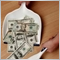41
47 976

### Introduction

This article will introduce MetaTrader 5 to ENCOG - advanced neural network and machine learning framework developed by Heaton Research. There are previously described methods I know of that enable MetaTrader to use machine learning techniques: FANN, NeuroSolutions, Matlab and NeuroShell. I hope that ENCOG will be a complementary solution since it is a robust and well designed code.

Why I chose ENCOG? There are a few reasons.

1. ENCOG is used in two other commercial trading software packages. One is based on C#, second on JAVA. This means it has already been tested to predict financial timeseries data.
2. ENCOG is free and open source software. If you would like to see what is going on inside a neural network you can browse the source code. This is what I actually did to understand parts of the timeseries prediciton problem. C# is clean and easy to understand programming language.
3. ENCOG is very well documented. Mr Heaton, founder of Heaton Research provides a free online course on Neural Networks, Machine Learning and using ENCOG to predict future data. I went through many of his lessons before writing this article. They helped me to understand Artificial Neural Networks a lot. Additionaly there are e-books about programming ENCOG in JAVA and C# on Heaton Research website. Full ENCOG documentation is available online.
4. ENCOG is not a dead project. As the time of writing this article ENCOG 2.6 is still under development. ENCOG 3.0 roadmap has recently been published.
5. ENCOG is robust. It is well designed, can use multiple CPU cores and multithreading to speed up neural network calculations. Parts of the code start to be ported for OpenCL - GPU enabled calculations.
6. ECNOG currently supported features:

Machine Learning Types

Neural Network Architectures

Training Techniques
Activation Functions
Randomization Techniques
• Range Randomization
• Gaussian Random Numbers
• Fan-In
• Nguyen-Widrow

Planned features:

As you can see this is quite a long feature list.

This introductory article focuses on feed forward Neural Network architecture with Resilient Propagation (RPROP) training. It also covers basics of data preparation - timeboxing and normalization for temporal timeseries prediciton.

The knowledge that enabled me to write this article is based on tutorials available on Heaton Research website and very recent articles on predicition of financial timeseries in NinjaTrader. Please note that ENCOG is JAVA and C# based. This article would not be possible to write without my previous work: Exposing C# code to MQL5 using unmanaged exports. This solution enabled to use C# DLL as a bridge between Metatrader 5 indicator and ENCOG timeseries predictor.

### 1. Using Technical Indicators Values as Inputs for a Neural Network

The Artificial Neural Network is a human-engineered algorithm that tries to emulate brain's neural network.

There are various types of neural algorithms available, and there exists a variety of neural network architectures. The research field is so broad that there are whole books devoted to a single type of a neural network. Since such details are out of scope of this article I can only recommend going through Heaton Research tutorials or reading a book on the subject. I will concentrate on inputs and outputs of the feed forward neural network and try to describe the practical example of financial timeseries prediction.

In order to start forecasting financial timeseries we have to think what should we provide to neural network and what could we expect in return. In most abstract black-box thinking we achieve profit or loss by taking long or short positions on the contract of a given security and closing the deal after some time.

By the observation of past prices of a security and values of the technical indicators we try to predict future sentiment or direction of the prices in order to buy or sell a contract and make sure our decision is not taken by flipping a coin. The situation looks more less like on the figure below: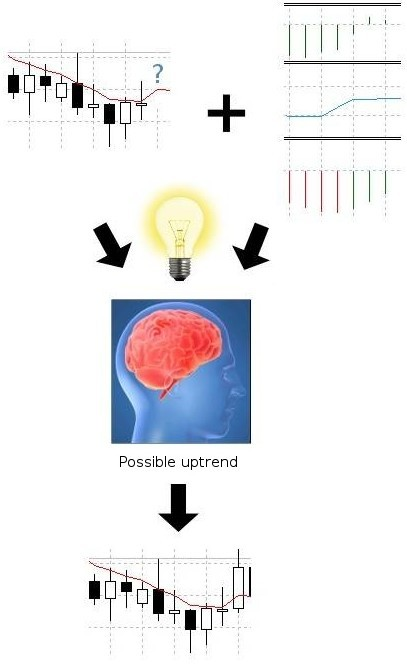Figure 1. Forecasting financial timeseries using technical indicators

We will try to achieve the same with artificial intelligence. The neural network will try to recognize indicator values and decide whether there is a chance that price will go up or down. How do we achieve that? Since we will be forecasting financial timeseries using the feed forward neural network architecture, I think we need to make an introduction to its architecture.

The feed forward neural network consists of neurons that are grouped in layers. There must be minimum 2 layers: an input layer that contains input neurons and an output layer that contains output neurons. There can be also hidden layers that are between the input and output layers. Input layer can be simply thought of an array of double values and output layer can consist of one or more neurons that also form an array of double values. Please see the figure below: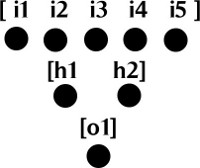Figure 2. Feedforward neural network layers

The connections between neurons were not drawn in order to simplify the drawing. Each neuron from the input layer is connected to a neuron in the hidden layer. Each neuron from the hidden layer is connected to a neuron in the output layer.

Each connection has its weight, which is also a double value and activation function with a threshold, that is responsible for activating a neuron and passing the information to the next neuron. This is why it is called a 'feed forward' network - information based on outputs of activated neurons is fed forward from one layer to another layer of neurons. For detailed introductory videos on feed forward neural networks you may want to visit the following links:

After you learn about neural network architecture and its mechanisms you may still be puzzled.

The main problems are:

1. What data shall we feed to a neural network?
2. How shall we feed it?
3. How to prepare input data for a neural network?
4. How to choose neural network architecture? How many input neurons, hidden neurons and output neurons do we need?
5. How to train the network?
6. What expect to be the output?

### 2. What Data to Feed the Neural Network with

Since we are dealing with financial predictions based on indicator outputs we should feed the network with indicators output values. For this article I chose Stochastic %K, Stochastic Slow %D, and Williams %R as inputs.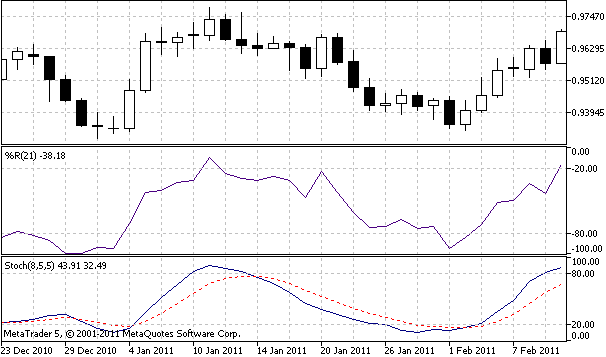Figure 3. Technical indicators used for prediction

In order to extract values of the indicators we can use iStochastic and iWPR MQL5 functions:

```double StochKArr[], StochDArr[], WilliamsRArr[];

ArraySetAsSeries(StochKArr, true);
ArraySetAsSeries(StochDArr, true);
ArraySetAsSeries(WilliamsRArr, true);

int hStochastic = iStochastic(Symbol(), Period(), 8, 5, 5, MODE_EMA, STO_LOWHIGH);
int hWilliamsR = iWPR(Symbol(), Period(), 21);

CopyBuffer(hStochastic, 0, 0, bufSize, StochKArr);
CopyBuffer(hStochastic, 1, 0, bufSize, StochDArr);
CopyBuffer(hWilliamsR, 0, 0, bufSize, WilliamsRArr);
```

After this code is executed three arrays StochKArr, StochDArr and WilliamsRArr should be filled with indicators output values. Depending on training sample size this may up to a few thousand values. Please have in mind that those two indicators were chosen only for educational purposes.

You are encouraged to experiment with any indicators you find appropriate for prediction. You may want to feed the network with gold and oil prices to predict stock indexes or you may use correlated forex pairs to predict another currency pair.

### 3. Timeboxing Input Data

Having gathered input data from several indicators we need to 'timebox' the input before feeding it into the neural network. Timeboxing is a technique that allows to present inputs for the network as moving slices of data. You can imagine a moving box of input data moving forward on time axis. There are basically two steps involved in this procedure:

1. Gathering input data from each indicator buffer. We need to copy INPUT_WINDOW number of elements from starting position towards the future. Input window is the number of bars used for prediction.Figure 4. Gathering input window data from indicator buffer

As you can see in the above example INPUT_WINDOW equals 4 bars and we copied elements into I1 array. I1 is first element I1 is the last one. Similarly data has to be copied from other indicators into INPUT_WINDOW size arrays. This figure is valid for timeseries arrays with AS_SERIES flag set to true.

2. Combining INPUT_WINDOW arrays into one array that is fed into neural network input layer.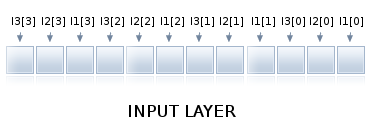Figure 5. Timeboxed input window arrays

There are 3 indicators, at first we take first value of each indicator, then second value of each indicator, and we continue until input window is filled like on the figure above. Such array combined from indicators outputs can be fed to the input layer of our neural network. When a new bar arrives, data is sliced by one element and whole procedure is repeated. If you are interested in more details about preparing the data for prediction you may also want to view a video on the subject.

### 4. Normalizing Input Data

In order to make neural network effective we must normalize the data. This is needed for correct calculation of activation functions. Normalization is a mathematical process that converts data into range 0..1 or -1..1. Normalized data can be denormalized, in other words converted back into original range.

Denormalization is needed to decode neural network output to a human readable form. Thankfully ENCOG takes care of normalization and denormalization, therefore there is no need to implement it. If you are curious how it works you may analyze the following code:

```/**
* Normalize the specified value.
* @param value The value to normalize.
* @return The normalized value.
*/
public static double normalize(final int value) {
return ((value - INPUT_LOW)
/ (INPUT_HIGH - INPUT_LOW))
* (OUTPUT_HIGH - OUTPUT_LOW) + OUTPUT_LOW;
}

/**
* De-normalize the specified value.
* @param value The value to denormalize.
* @return The denormalized value.
*/
public static double deNormalize(final double data) {
double result = ((INPUT_LOW - INPUT_HIGH) * data - OUTPUT_HIGH
* INPUT_LOW + INPUT_HIGH * OUTPUT_LOW)
/ (OUTPUT_LOW - OUTPUT_HIGH);
return result;
}
```

and read an article on normalization for further details.

### 5. Choosing network architecture and number of neurons

For a newbie on the subject choosing correct network architecture is a hard part. In this article I am restricting the feedfoward neural network architecture to three layers: an input layer, one hidden layer and an output layer. You are free to experiment with greater number of layers.

For input and output layer we will be able to accurately count the number of neurons needed. For a hidden layer we will be trying to minimize neural network error by using a forward selection algorithm. You are encouraged to use other methods; there may be some genetic algorithms for calculating the number of neurons.

Another method used by ENCOG is called backward selection algorithm or pruning, basically it is evaluating connections between the layers and removing hidden neurons with zero weighted connections, you may also want to try it out.

5.1. Input neurons layer

Due to timeboxing the number of neurons in the input layer should be equal to number of indicators times number of bars used to predict the next bar. If we use 3 indicators as inputs and input window size is equal to 6 bars, the input layer will consist of 18 neurons. Input layer is fed with data prepared by timeboxing.

5.2. Hidden neurons layer

The number of hidden networks must be estimated based on trained neural network performance. There is no straightforward mathematical equation for a number of hidden neurons. Before writing the article I used multiple trial-and-error approaches and I found an algorihtm on Heaton Research website that helps to understand the forward selection algorithm:Figure 6. Forward selection algorithm for number of hidden neurons

5.3. Output neurons layer

For our purposes the number of output neurons is the number of bars we are trying to predict. Please remember that the bigger number of hidden and output neurons, the longer takes the network to train. In this article I am trying to predict one bar in the future, therefore the output layer consists of one neuron.

### 6. Exporting Training Data from MetaTrader 5 to ENCOG

Encog accepts CSV file for neural network training.

I looked at the file format exported from other trading software to ENCOG and implemented MQL5 script that prepares the same file format for training. I will present at first exporting one indicator and continue later with multiple indicators.

The first line of data is a comma separated header:

`DATE,TIME,CLOSE,Indicator_Name1,Indicator_Name2,Indicator_Name3`

First three columns contain, date, time and close values, next columns contain indicator names. Next rows of the training file should contain comma separated data, indicator values should be written in scientific format:

`20110103,0000,0.93377000,-7.8970208860e-002`

```//+------------------------------------------------------------------+
//|                                                ExportToEncog.mq5 |
//|                                                http:/Investeo.pl |
//+------------------------------------------------------------------+
#property version   "1.00"
//+------------------------------------------------------------------+
//| Script program start function                                    |
//+------------------------------------------------------------------+

// Export Indicator values for NN training by ENCOG
extern string IndExportFileName = "mt5export.csv";
extern int  trainSize = 400;
extern int  maPeriod = 210;

MqlRates srcArr[];
double expBullsArr[];

void OnStart()
{
//---
ArraySetAsSeries(srcArr, true);
ArraySetAsSeries(expBullsArr, true);

int copied = CopyRates(Symbol(), Period(), 0, trainSize, srcArr);

if (copied!=trainSize) { Print("Not enough data for " + Symbol()); return; }

int hBullsPower = iBullsPower(Symbol(), Period(), maPeriod);

CopyBuffer(hBullsPower, 0, 0, trainSize, expBullsArr);

int hFile = FileOpen(IndExportFileName, FILE_CSV | FILE_ANSI | FILE_WRITE | FILE_REWRITE, ",", CP_ACP);

FileWriteString(hFile, "DATE,TIME,CLOSE,BullsPower\n");

Print("Exporting indicator data to " + IndExportFileName);

for (int i=trainSize-1; i>=0; i--)
{
string candleDate = TimeToString(srcArr[i].time, TIME_DATE);
StringReplace(candleDate,".","");
string candleTime = TimeToString(srcArr[i].time, TIME_MINUTES);
StringReplace(candleTime,":","");
FileWrite(hFile, candleDate, candleTime, DoubleToString(srcArr[i].close), DoubleToString(expBullsArr[i], -10));
}

FileClose(hFile);

Print("Indicator data exported.");
}
//+------------------------------------------------------------------+
```

Result file that can be used for training should look like the following output:

```DATE,TIME,CLOSE,BullsPower
20110103,0000,0.93377000,-7.8970208860e-002
20110104,0000,0.94780000,-6.4962292188e-002
20110105,0000,0.96571000,-4.7640374727e-002
20110106,0000,0.96527000,-4.4878854587e-002
20110107,0000,0.96697000,-4.6178012364e-002
20110110,0000,0.96772000,-4.2078647318e-002
20110111,0000,0.97359000,-3.6029181466e-002
20110112,0000,0.96645000,-3.8335729509e-002
20110113,0000,0.96416000,-3.7054869514e-002
20110114,0000,0.96320000,-4.4259373120e-002
20110117,0000,0.96503000,-4.4835729773e-002
20110118,0000,0.96340000,-4.6420936126e-002
20110119,0000,0.95585000,-4.6868984125e-002
20110120,0000,0.96723000,-4.2709941621e-002
20110121,0000,0.95810000,-4.1918330800e-002
20110124,0000,0.94873000,-4.7722659418e-002
20110125,0000,0.94230000,-5.7111591557e-002
20110126,0000,0.94282000,-6.2231529077e-002
20110127,0000,0.94603000,-5.9997865295e-002
20110128,0000,0.94165000,-6.0378312069e-002
20110131,0000,0.94414000,-6.2038328069e-002
20110201,0000,0.93531000,-6.0710334438e-002
20110202,0000,0.94034000,-6.1446445012e-002
20110203,0000,0.94586000,-5.2580791504e-002
20110204,0000,0.95496000,-4.5246755566e-002
20110207,0000,0.95730000,-4.4439392954e-002
```

Going back to the original article example with Stochastic and Williams' R indicators we need to export three coma separated columns, each column contains separate indicator values, therefore we need to expand the file and add additional buffers:

```//+------------------------------------------------------------------+
//|                                                ExportToEncog.mq5 |
//|                                                http:/Investeo.pl |
//+------------------------------------------------------------------+
#property version   "1.00"
//+------------------------------------------------------------------+
//| Script program start function                                    |
//+------------------------------------------------------------------+

// Export Indicator values for NN training by ENCOG
extern string IndExportFileName = "mt5export.csv";
extern int  trainSize = 2000;

MqlRates srcArr[];
double StochKArr[], StochDArr[], WilliamsRArr[];

void OnStart()
{
//---
ArraySetAsSeries(srcArr, true);
ArraySetAsSeries(StochKArr, true);
ArraySetAsSeries(StochDArr, true);
ArraySetAsSeries(WilliamsRArr, true);

int copied = CopyRates(Symbol(), Period(), 0, trainSize, srcArr);

if (copied!=trainSize) { Print("Not enough data for " + Symbol()); return; }

int hStochastic = iStochastic(Symbol(), Period(), 8, 5, 5, MODE_EMA, STO_LOWHIGH);
int hWilliamsR = iWPR(Symbol(), Period(), 21);

CopyBuffer(hStochastic, 0, 0, trainSize, StochKArr);
CopyBuffer(hStochastic, 1, 0, trainSize, StochDArr);
CopyBuffer(hWilliamsR, 0, 0, trainSize, WilliamsRArr);

int hFile = FileOpen(IndExportFileName, FILE_CSV | FILE_ANSI | FILE_WRITE | FILE_REWRITE, ",", CP_ACP);

FileWriteString(hFile, "DATE,TIME,CLOSE,StochK,StochD,WilliamsR\n");

Print("Exporting indicator data to " + IndExportFileName);

for (int i=trainSize-1; i>=0; i--)
{
string candleDate = TimeToString(srcArr[i].time, TIME_DATE);
StringReplace(candleDate,".","");
string candleTime = TimeToString(srcArr[i].time, TIME_MINUTES);
StringReplace(candleTime,":","");
FileWrite(hFile, candleDate, candleTime, DoubleToString(srcArr[i].close),
DoubleToString(StochKArr[i], -10),
DoubleToString(StochDArr[i], -10),
DoubleToString(WilliamsRArr[i], -10)
);
}

FileClose(hFile);

Print("Indicator data exported.");
}
//+------------------------------------------------------------------+
```

Result file should have all indicator values:

```DATE,TIME,CLOSE,StochK,StochD,WilliamsR
20030707,0000,1.37370000,7.1743119266e+001,7.2390220187e+001,-6.2189054726e-001
20030708,0000,1.36870000,7.5140977444e+001,7.3307139273e+001,-1.2500000000e+001
20030709,0000,1.35990000,7.3831775701e+001,7.3482018082e+001,-2.2780373832e+001
20030710,0000,1.36100000,7.1421933086e+001,7.2795323083e+001,-2.1495327103e+001
20030711,0000,1.37600000,7.5398313027e+001,7.3662986398e+001,-3.9719626168e+000
20030714,0000,1.37370000,7.0955352856e+001,7.2760441884e+001,-9.6153846154e+000
20030715,0000,1.38560000,7.4975891996e+001,7.3498925255e+001,-2.3890784983e+000
20030716,0000,1.37530000,7.5354107649e+001,7.4117319386e+001,-2.2322435175e+001
20030717,0000,1.36960000,7.1775345074e+001,7.3336661282e+001,-3.0429594272e+001
20030718,0000,1.36280000,5.8474576271e+001,6.8382632945e+001,-3.9778325123e+001
20030721,0000,1.35400000,4.3498596819e+001,6.0087954237e+001,-5.4946524064e+001
20030722,0000,1.36130000,2.9036761284e+001,4.9737556586e+001,-4.5187165775e+001
20030723,0000,1.34640000,1.6979405034e+001,3.8818172735e+001,-6.5989159892e+001
20030724,0000,1.34680000,1.0634573304e+001,2.9423639592e+001,-7.1555555556e+001
20030725,0000,1.34400000,9.0909090909e+000,2.2646062758e+001,-8.7500000000e+001
20030728,0000,1.34680000,1.2264922322e+001,1.9185682613e+001,-8.2705479452e+001
20030729,0000,1.35250000,1.4960629921e+001,1.7777331716e+001,-7.2945205479e+001
20030730,0000,1.36390000,2.7553336360e+001,2.1035999930e+001,-5.3979238754e+001
20030731,0000,1.36990000,4.3307839388e+001,2.8459946416e+001,-4.3598615917e+001
20030801,0000,1.36460000,5.6996412096e+001,3.7972101643e+001,-5.2768166090e+001
20030804,0000,1.34780000,5.7070193286e+001,4.4338132191e+001,-8.1833910035e+001
20030805,0000,1.34770000,5.3512705531e+001,4.7396323304e+001,-8.2006920415e+001
20030806,0000,1.35350000,4.4481132075e+001,4.6424592894e+001,-7.1972318339e+001
20030807,0000,1.35020000,3.3740028156e+001,4.2196404648e+001,-7.7681660900e+001
20030808,0000,1.35970000,3.0395426394e+001,3.8262745230e+001,-6.1245674740e+001
20030811,0000,1.35780000,3.4155781326e+001,3.6893757262e+001,-6.4532871972e+001
20030812,0000,1.36880000,4.3488943489e+001,3.9092152671e+001,-4.5501730104e+001
20030813,0000,1.36690000,5.1160443996e+001,4.3114916446e+001,-4.8788927336e+001
20030814,0000,1.36980000,6.2467599793e+001,4.9565810895e+001,-2.5629290618e+001
20030815,0000,1.37150000,6.9668246445e+001,5.6266622745e+001,-2.1739130435e+001
20030818,0000,1.38910000,7.9908906883e+001,6.4147384124e+001,-9.2819614711e+000
```

You can modify the second example to easily produce a script that will suit your needs.

### 7. Neural Network Training

Training of the network has already been prepared in C# by Heaton Research. ENCOG 2.6 implements Encog.App.Quant namespace that is a basis for financial timeseries prediction. The training script is very flexible can be easily adjusted to any number of input indicators. You should only change MetaTrader 5 directory location in DIRECTORY constant.

Network architecture and training parameters can be easily customized by changing the following variables:

```        /// <summary>
/// The size of the input window.  This is the number of bars used to predict the next bar.
/// </summary>
public const int INPUT_WINDOW = 6;

/// <summary>
/// The number of bars forward we are trying to predict.  This is usually just 1 bar.  The future indicator used in step 1 may
/// well look more forward into the future.
/// </summary>
public const int PREDICT_WINDOW = 1;

/// <summary>
/// The number of bars forward to look for the best result.
/// </summary>
public const int RESULT_WINDOW = 5;

/// <summary>
/// The number of neurons in the first hidden layer.
/// </summary>
public const int HIDDEN1_NEURONS = 12;

/// <summary>
/// The target error to train to.
/// </summary>
public const double TARGET_ERROR = 0.01;```

The code is very self explanatory, therefore the best will be to read it carefully:

```using System;
using System.Collections.Generic;
using System.Linq;
using System.Text;
using Encog.App.Quant.Normalize;
using Encog.Util.CSV;
using Encog.App.Quant.Indicators;
using Encog.App.Quant.Indicators.Predictive;
using Encog.App.Quant.Temporal;
using Encog.Neural.NeuralData;
using Encog.Neural.Data.Basic;
using Encog.Util.Simple;
using Encog.Neural.Networks;
using Encog.Neural.Networks.Layers;
using Encog.Engine.Network.Activation;
using Encog.Persist;

namespace NetworkTrainer
{
public class Program
{
/// <summary>
/// The directory that all of the files will be stored in.
/// </summary>
public const String DIRECTORY = "d:\\mt5\\MQL5\\Files\\";

/// <summary>
/// The input file that starts the whole process.  This file should be downloaded from NinjaTrader using the EncogStreamWriter object.
/// </summary>
public const String STEP1_FILENAME = DIRECTORY + "mt5export.csv";

/// <summary>
/// We apply a predictive future indicator and generate a second file, with the additional predictive field added.
/// </summary>
public const String STEP2_FILENAME = DIRECTORY + "step2_future.csv";

/// <summary>
/// Next the entire file is normalized and stored into this file.
/// </summary>
public const String STEP3_FILENAME = DIRECTORY + "step3_norm.csv";

/// <summary>
/// The file is time-boxed to create training data.
/// </summary>
public const String STEP4_FILENAME = DIRECTORY + "step4_train.csv";

/// <summary>
/// Finally, the trained neural network is written to this file.
/// </summary>
public const String STEP5_FILENAME = DIRECTORY + "step5_network.eg";

/// <summary>
/// The size of the input window.  This is the number of bars used to predict the next bar.
/// </summary>
public const int INPUT_WINDOW = 6;

/// <summary>
/// The number of bars forward we are trying to predict.  This is usually just 1 bar.  The future indicator used in step 1 may
/// well look more forward into the future.
/// </summary>
public const int PREDICT_WINDOW = 1;

/// <summary>
/// The number of bars forward to look for the best result.
/// </summary>
public const int RESULT_WINDOW = 5;

/// <summary>
/// The number of neurons in the first hidden layer.
/// </summary>
public const int HIDDEN1_NEURONS = 12;

/// <summary>
/// The target error to train to.
/// </summary>
public const double TARGET_ERROR = 0.01;

static void Main(string[] args)
{
// Step 1: Create future indicators
Console.WriteLine("Step 1: Analyze MT5 Export & Create Future Indicators");
ProcessIndicators ind = new ProcessIndicators();
ind.Analyze(STEP1_FILENAME, true, CSVFormat.DECIMAL_POINT);
int externalIndicatorCount = ind.Columns.Count - 3;
ind.Process(STEP2_FILENAME);
Console.WriteLine("External indicators found: " + externalIndicatorCount);

// Step 2: Normalize
Console.WriteLine("Step 2: Create Future Indicators");
EncogNormalize norm = new EncogNormalize();
norm.Analyze(STEP2_FILENAME, true, CSVFormat.ENGLISH);
norm.Stats.Action = NormalizationDesired.PassThrough; // Date
norm.Stats.Action = NormalizationDesired.PassThrough; // Time

norm.Stats.Action = NormalizationDesired.Normalize; // Close
norm.Stats.Action = NormalizationDesired.Normalize; // Stoch K
norm.Stats.Action = NormalizationDesired.Normalize; // Stoch Dd
norm.Stats.Action = NormalizationDesired.Normalize; // WilliamsR

norm.Stats.Action = NormalizationDesired.Normalize; // best return [RESULT_WINDOW]

norm.Normalize(STEP3_FILENAME);

// neuron counts
int inputNeurons = INPUT_WINDOW * externalIndicatorCount;
int outputNeurons = PREDICT_WINDOW;

// Step 3: Time-box
Console.WriteLine("Step 3: Timebox");
TemporalWindow window = new TemporalWindow();
window.Analyze(STEP3_FILENAME, true, CSVFormat.ENGLISH);
window.InputWindow = INPUT_WINDOW;
window.PredictWindow = PREDICT_WINDOW;
int index = 0;
window.Fields[index++].Action = TemporalType.Ignore; // date
window.Fields[index++].Action = TemporalType.Ignore; // time
window.Fields[index++].Action = TemporalType.Ignore; // close
for(int i=0;i<externalIndicatorCount;i++)
window.Fields[index++].Action = TemporalType.Input; // external indicators
window.Fields[index++].Action = TemporalType.Predict; // PredictBestReturn

window.Process(STEP4_FILENAME);

// Step 4: Train neural network
Console.WriteLine("Step 4: Train");
outputNeurons, true, CSVFormat.ENGLISH);

BasicNetwork network = new BasicNetwork();
network.Structure.FinalizeStructure();
network.Reset();

//EncogUtility.TrainToError(network, training, TARGET_ERROR);
EncogUtility.TrainConsole(network, training, 3);

// Step 5: Save neural network and stats
EncogMemoryCollection encog = new EncogMemoryCollection();
encog.Save(STEP5_FILENAME);
}
}
}```

You may notice that I commented out one line and changed training function from EncogUtility.TrainToError() to EncogUtility.TrainConsole()

`EncogUtility.TrainConsole(network, training, 3);`

TrainConsole method specifies a number of minutes to train the network. In the example I train the network for three minutes. Depending on complexity of the network and size of the training data, trainging the network may take a few minutes, hours or even days. I recommend to read more on the error calculation and training algorithms on Heaton Research website or any other book on the subject.

EncogUtility.TrainToError() methods stops training the network after a target network error is achieved. You may comment EncongUtiliy.TrainConsole() and uncomment EncogUtility.TrainToError() to train the network up to a desired error like in the original example

```EncogUtility.TrainToError(network, training, TARGET_ERROR);
```

Please notice that sometimes network cannot be trained to a certain error because number of neurons may be too small.

### 8. Using Trained Neural Network to Build MetaTrader 5 Neural Indicator

Trained network can be used by a neural network indicator that will try to predict the best return on investment.

The ENCOG neural indicator for MetaTrader 5 consists of two parts. One part is written in MQL5 and it basically takes the same indicators as the ones the network was trained with and feeds the network with input window indicator values. The second part is written in C# and it timeboxes input data and returns neural network output to MQL5. The C# indicator part is based on my previous article on Exposing C# code to MQL5.

```using System;
using System.Collections.Generic;
using System.Text;
using RGiesecke.DllExport;
using System.Runtime.InteropServices;
using Encog.Neural.Networks;
using Encog.Persist;
using Encog.App.Quant.Normalize;
using Encog.Neural.Data;
using Encog.Neural.Data.Basic;

namespace EncogNeuralIndicatorMT5DLL
{

public class NeuralNET
{
private EncogMemoryCollection encog;
public BasicNetwork network;
public NormalizationStats stats;

public NeuralNET(string nnPath)
{
initializeNN(nnPath);
}

public void initializeNN(string nnPath)
{
try
{
encog = new EncogMemoryCollection();
network = (BasicNetwork)encog.Find("network");
stats = (NormalizationStats)encog.Find("stat");
}
catch (Exception e)
{
Console.WriteLine(e.StackTrace);
}
}
};

class UnmanagedExports
{

static NeuralNET neuralnet;

[DllExport("initializeTrainedNN", CallingConvention = CallingConvention.StdCall)]
static int initializeTrainedNN([MarshalAs(UnmanagedType.LPWStr)]string nnPath)
{
neuralnet = new NeuralNET(nnPath);

if (neuralnet.network != null) return 0;
else return -1;
}

[DllExport("computeNNIndicator", CallingConvention = CallingConvention.StdCall)]
public static int computeNNIndicator([MarshalAs(UnmanagedType.LPArray, SizeParamIndex = 3)] double[] t1,
[MarshalAs(UnmanagedType.LPArray, SizeParamIndex = 3)] double[] t2,
[MarshalAs(UnmanagedType.LPArray, SizeParamIndex = 3)] double[] t3,
int len,
[In, Out, MarshalAs(UnmanagedType.LPArray, SizeParamIndex = 5)] double[] result,
int rates_total)
{
INeuralData input = new BasicNeuralData(3 * len);

int index = 0;
for (int i = 0; i <len; i++)
{
input[index++] = neuralnet.stats.Normalize(t1[i]);
input[index++] = neuralnet.stats.Normalize(t2[i]);
input[index++] = neuralnet.stats.Normalize(t3[i]);
}

INeuralData output = neuralnet.network.Compute(input);
double d = output;
d = neuralnet.stats.DeNormalize(d);
result[rates_total-1]=d;

return 0;
}
}
}
```

If you would like to use any other number of indicators than three you need to change computeNNIndicator() method to suit your needs.

``` [DllExport("computeNNIndicator", CallingConvention = CallingConvention.StdCall)]
public static int computeNNIndicator([MarshalAs(UnmanagedType.LPArray, SizeParamIndex = 3)] double[] t1,
[MarshalAs(UnmanagedType.LPArray, SizeParamIndex = 3)] double[] t2,
[MarshalAs(UnmanagedType.LPArray, SizeParamIndex = 3)] double[] t3,
int len,
[In, Out, MarshalAs(UnmanagedType.LPArray, SizeParamIndex = 5)] double[] result,
int rates_total)```

In this case three first input parameters are tables that contain indicator input values, fourth parameter is input window length.

SizeParamIndex = 3 points to input window length variable, as input variables count is increased from 0 onwards. Fifth parameter is a table that contains neural network results.

MQL5 indicator part needs to import a C# EncogNNTrainDLL.dll and use initializeTrainedNN() and computeNNIndicator() functions exported from the dll.

```//+------------------------------------------------------------------+
//|                                         NeuralEncogIndicator.mq5 |
//|                                                http:/Investeo.pl |
//+------------------------------------------------------------------+
#property version   "1.00"
#property indicator_separate_window

#property indicator_plots 1
#property indicator_buffers 1
#property indicator_color1 Blue
#property indicator_type1 DRAW_LINE
#property indicator_style1 STYLE_SOLID
#property indicator_width1  2

#import "EncogNNTrainDLL.dll"
int initializeTrainedNN(string nnFile);
int computeNNIndicator(double& ind1[], double& ind2[],double& ind3[], int size, double& result[], int rates);
#import

int INPUT_WINDOW = 6;
int PREDICT_WINDOW = 1;

double ind1Arr[], ind2Arr[], ind3Arr[];
double neuralArr[];

int hStochastic;
int hWilliamsR;

int hNeuralMA;
//+------------------------------------------------------------------+
//| Custom indicator initialization function                         |
//+------------------------------------------------------------------+
int OnInit()
{
//--- indicator buffers mapping
SetIndexBuffer(0, neuralArr, INDICATOR_DATA);

PlotIndexSetInteger(0, PLOT_SHIFT, 1);

ArrayResize(ind1Arr, INPUT_WINDOW);
ArrayResize(ind2Arr, INPUT_WINDOW);
ArrayResize(ind3Arr, INPUT_WINDOW);

ArrayInitialize(neuralArr, 0.0);

ArraySetAsSeries(ind1Arr, true);
ArraySetAsSeries(ind2Arr, true);
ArraySetAsSeries(ind3Arr, true);

ArraySetAsSeries(neuralArr, true);

hStochastic = iStochastic(NULL, 0, 8, 5, 5, MODE_EMA, STO_LOWHIGH);
hWilliamsR = iWPR(NULL, 0, 21);

Print(TerminalInfoString(TERMINAL_DATA_PATH)+"\\MQL5\Files\step5_network.eg");
initializeTrainedNN(TerminalInfoString(TERMINAL_DATA_PATH)+"\\MQL5\Files\step5_network.eg");

//---
return(0);
}
//+------------------------------------------------------------------+
//| Custom indicator iteration function                              |
//+------------------------------------------------------------------+
int OnCalculate(const int rates_total,
const int prev_calculated,
const datetime& time[],
const double& open[],
const double& high[],
const double& low[],
const double& close[],
const long& tick_volume[],
const long& volume[],
{
//---
int calc_limit;

if(prev_calculated==0) // First execution of the OnCalculate() function after the indicator start
calc_limit=rates_total-34;
else calc_limit=rates_total-prev_calculated;

ArrayResize(neuralArr, rates_total);

for (int i=0; i<calc_limit; i++)
{
CopyBuffer(hStochastic, 0, i, INPUT_WINDOW, ind1Arr);
CopyBuffer(hStochastic, 1, i, INPUT_WINDOW, ind2Arr);
CopyBuffer(hWilliamsR,  0, i, INPUT_WINDOW, ind3Arr);

computeNNIndicator(ind1Arr, ind2Arr, ind3Arr, INPUT_WINDOW, neuralArr, rates_total-i);
}

//Print("neuralArr = " + neuralArr);

//--- return value of prev_calculated for next call
return(rates_total);
}
//+------------------------------------------------------------------+
```

Please see the indicator output trained on USDCHF daily data and Stochastic and Williams %R indicators:Figure 7. Neural Encog indicator

The indicator shows predicted best return on investment on the next bar.

You may have noticed I shifted the indicator one bar in the future:

`PlotIndexSetInteger(0, PLOT_SHIFT, 1);`

This is to indicate that the indicator is a predictive one. Since we built a neural indicator we are ready to build an Expert Advisor based on the indicator.

### 9. Expert Advisor Based on a Neural Indicator

The Expert Advisor takes neural indicator output and decides whether to buy or sell a security. My first impression was that it should buy whenever indicator is above zero and sell when it is below zero, meaning buy when best return prediction on a given time window is positive and sell when best return prediction is negative.

After some initial testing it turned out that the performance could be better, so I introduced 'strong uptrend' and 'strong downtrend' variables, meaning that there is no reason to exit the trade when we are in a strong trend according to famous 'trend is you friend' rule.

Additionally I was advised on Heaton Research forum to use ATR for moving stop losses, so I used Chandelier ATR indicator I found on MQL5 forum. It indeed increased equity gain while backtesting. I am pasting the source code of the Expert Advisor below.

```//+------------------------------------------------------------------+
//|                                                http:/Investeo.pl |
//+------------------------------------------------------------------+
#property version   "1.00"

double neuralArr[];

double trend;
double Lots=0.3;

int INPUT_WINDOW=8;

int hNeural,hChandelier;

//+------------------------------------------------------------------+
//+------------------------------------------------------------------+

//+------------------------------------------------------------------+
//| Expert initialization function                                   |
//+------------------------------------------------------------------+
int OnInit()
{
//---
ArrayResize(neuralArr,INPUT_WINDOW);
ArraySetAsSeries(neuralArr,true);
ArrayInitialize(neuralArr,0.0);

hNeural=iCustom(Symbol(),Period(),"NeuralEncogIndicator");
Print("hNeural = ",hNeural,"  error = ",GetLastError());

if(hNeural<0)
{
Print("The creation of ENCOG indicator has failed: Runtime error =",GetLastError());
//--- forced program termination
return(-1);
}
else  Print("ENCOG indicator initialized");

hChandelier=iCustom(Symbol(),Period(),"Chandelier");
Print("hChandelier = ",hChandelier,"  error = ",GetLastError());

if(hChandelier<0)
{
Print("The creation of Chandelier indicator has failed: Runtime error =",GetLastError());
//--- forced program termination
return(-1);
}
else  Print("Chandelier indicator initialized");
//---
return(0);
}
//+------------------------------------------------------------------+
//| Expert deinitialization function                                 |
//+------------------------------------------------------------------+
void OnDeinit(const int reason)
{
//---
}
//+------------------------------------------------------------------+
//| Expert tick function                                             |
//+------------------------------------------------------------------+
void OnTick()
{
//---
long tickCnt;
int ticks=CopyTickVolume(Symbol(),0,0,1,tickCnt);
if(tickCnt==1)
{
if(!CopyBuffer(hNeural,0,0,INPUT_WINDOW,neuralArr)) { Print("Copy1 error"); return; }

// Print("neuralArr = "+neuralArr+"neuralArr = "+neuralArr+"neuralArr = "+neuralArr);
trend=0;

if(neuralArr<0 && neuralArr>0) trend=-1;
if(neuralArr>0 && neuralArr<0) trend=1;

}
}
//+------------------------------------------------------------------+
//| Tester function                                                  |
//+------------------------------------------------------------------+
double OnTester()
{
//---

//---
return(0.0);
}
//+------------------------------------------------------------------+

{
double bufChandelierUP;
double bufChandelierDN;

double bufMA;

ArraySetAsSeries(bufChandelierUP,true);
ArraySetAsSeries(bufChandelierUP,true);

ArraySetAsSeries(bufMA,true);

CopyBuffer(hChandelier,0,0,2,bufChandelierUP);
CopyBuffer(hChandelier,1,0,2,bufChandelierDN);

MqlRates rates[];
ArraySetAsSeries(rates,true);
int copied=CopyRates(Symbol(),PERIOD_CURRENT,0,3,rates);

bool strong_uptrend=neuralArr>0 && neuralArr>0 && neuralArr>0 &&
neuralArr>0 && neuralArr>0 && neuralArr>0 &&
neuralArr>0 && neuralArr>0;
bool strong_downtrend=neuralArr<0 && neuralArr<0 && neuralArr<0 &&
neuralArr<0 && neuralArr<0 && neuralArr<0 &&
neuralArr<0 && neuralArr<0;

if(PositionSelect(_Symbol))
{
long type=PositionGetInteger(POSITION_TYPE);
bool close=false;

if(!(strong_uptrend) || (bufChandelierUP==EMPTY_VALUE)) close=true;
if((type==POSITION_TYPE_SELL) && (trend==1))
if(!(strong_downtrend) || (bufChandelierDN==EMPTY_VALUE))
close=true;
if(close)
{
}
{

if(copied>0)
{
{
if(bufChandelierUP!=EMPTY_VALUE)
}
if(type==POSITION_TYPE_SELL)
{
if(bufChandelierDN!=EMPTY_VALUE)
}
}
}
}

if((trend!=0) && (!PositionSelect(_Symbol)))
{
MqlTick tick;
MqlRates rates[];
ArraySetAsSeries(rates,true);
int copied=CopyRates(Symbol(),PERIOD_CURRENT,0,INPUT_WINDOW,rates);

if(copied>0)
{
if(SymbolInfoTick(_Symbol,tick)==true)
{
if(trend>0)
{
}
if(trend<0)
{
Print("Sell at "+tick.ask+" trend = "+trend+" neuralArr = "+neuralArr);
}
}
}
}

}
//+------------------------------------------------------------------+
```

The Expert Advisor was run on USDCHF currency D1 data. About 50% of the data was out of sample of the training.

### 10. Expert Advisor BackTesting Results

I am pasting the backtesting results below. The backtest was run from 2000.01.01 to 2011.03.26.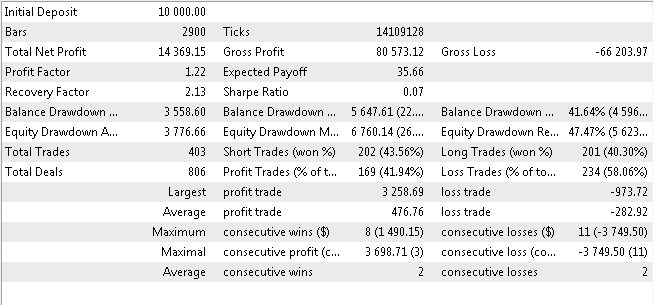Figure 8. Neural Expert Advisor backtesting resultsFigure 9. Neural Expert Advisor Balance/Equity backtesting graph

Please note that this performance may be totally different for orther timeframe and other securities.

Please treat this EA as educational one and make it a starting point for further research. My personal view is that the network could be retrained every certain period of time to make it more robust, maybe someone will or already found a good way to achieve that. Perhaps there is a better way to make Buy/Sell predictions based on a neural indicator. I encourage readers to experiment.

### Conclusion

In the following article I presented a way to build a neural predictive indicator and expert advisor based on that indicator with help of ENCOG machine learning framework. All source code, compiled binaries, DLLs and an exemplary trained network are attached to the article.

Because of "double DLL wrapping in .NET", the Cloo.dll, encog-core-cs.dll and log4net.dll files should be located in the folder of the client terminal.
The EncogNNTrainDLL.dll file should be located in \Terminal Data folder\MQL5\Libraries\ folder.

Attached files |
encogcsharp.zip (2202.77 KB)
files.zip (270.14 KB)
libraries.zip (321.62 KB)
experts.zip (1.56 KB)
scripts.zip (1.03 KB)
indicators.zip (2.24 KB)
Last comments | Go to discussion (41)| 15 Jun 2017 at 06:21
Can this work with MT4?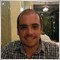| 5 Nov 2017 at 22:47
muttonman:

Awesome article investeo!

Just one problem, every time I run the EA on the simulator I get the following error...

2012.08.07 20:10:36 Core 1 2012.01.01 00:00:00   Access violation read to 0x00000000

I have followed the suggestions on this page. I still get the error.

Excellent article!! It's very clear for a beginner like me :)

I'm having the same problem with the attached encog version on Windows 10 X64.

Could anyone solve this? Any suggestions?

Thanks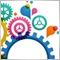| 17 Mar 2018 at 11:43

Anyway, is there anyone, before congratulating with the authors, who tried the entire codes? I have serious doubts that we are really close to random guessing, as expected with Forex.

I run a LSTM as mentioned in the article, focussing not on regression (guessing the next values), but on binary classification: if the max_close in the next 5 future steps is greater than the actual, than class_0 otherwise class_1. In few words, I ran an LSTM to predict if the close in the next future goes up or down. My precision is close to random guessing and I am pretty sure also the precision of the RNN of this article goes close to random guessing. I am really curious about the performances of the RNN of this article. Can the authors share the confusion matrix.

I also ran a fuzzy classifier just classifying the future close the same as the current: it is not definitely a prediction! With that I was able to reach similar profit of this article.

In conclusion, I suspect that the results of the RNN of this article are really close to random guessing and I would like to ask the authors to share with us the direct performance of their RNN.

Anyway, the article is inspiring for further research.

Cheers,

Marco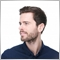| 17 May 2018 at 11:59
Which part of the code has used OpenCL? Please clarify.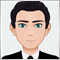| 23 Sep 2018 at 20:14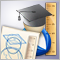How to Order an Expert Advisor and Obtain the Desired Result

How to write correctly the Requirement Specifications? What should and should not be expected from a programmer when ordering an Expert Advisor or an indicator? How to keep a dialog, what moments to pay special attention to? This article gives the answers to these, as well as to many other questions, which often don't seem obvious to many people.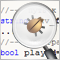Tracing, Debugging and Structural Analysis of Source Code

The entire complex of problems of creating a structure of an executed code and its tracing can be solved without serious difficulties. This possibility has appeared in MetaTrader 5 due to the new feature of the MQL5 language - automatic creation of variables of complex type of data (structures and classes) and their elimination when going out of local scope. The article contains the description of the methodology and the ready-made tool.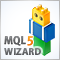MQL5 Wizard: New Version

The article contains descriptions of the new features available in the updated MQL5 Wizard. The modified architecture of signals allow creating trading robots based on the combination of various market patterns. The example contained in the article explains the procedure of interactive creation of an Expert Advisor.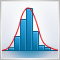Statistical Estimations

Estimation of statistical parameters of a sequence is very important, since most of mathematical models and methods are based on different assumptions. For example, normality of distribution law or dispersion value, or other parameters. Thus, when analyzing and forecasting of time series we need a simple and convenient tool that allows quickly and clearly estimating the main statistical parameters. The article shortly describes the simplest statistical parameters of a random sequence and several methods of its visual analysis. It offers the implementation of these methods in MQL5 and the methods of visualization of the result of calculations using the Gnuplot application.